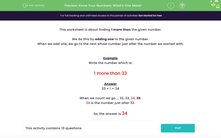# Know Your Numbers: Identify One More

In this worksheet, students will identify one number more than the given number (from a range between 0 and 100).Key stage:  KS 1

Curriculum topic:   Number: Number and Place Value

Curriculum subtopic:   Identify One More/One Less

Difficulty level:#### Worksheet Overview

This activity is about finding 1 more than a given number.We do this by adding 1 to the given number.

When we add 1, we go to the next whole number just after the number we started with.

Example 1

Write the number that is:

1 more than 33

33 + 1 = 34

When we count we go.... 32, 33, 34, 35.

34 is the number just after 33.

Example 2

Write the number that is:

1 more than 49

49 + 1 = 50

When we count we go ..... .47, 48, 49, 50

50 is the number just after 49.